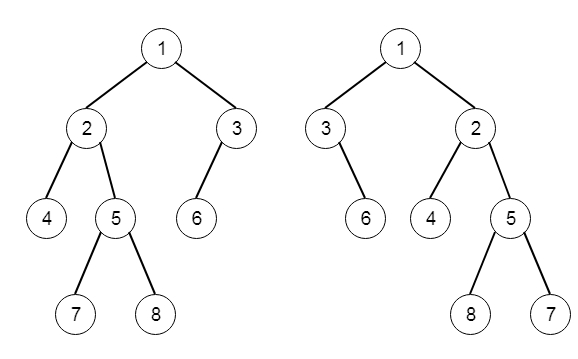# Flip Equivalent Binary Trees in C++

Suppose we have a binary tree. We have to flip the binary tree. The flip indicates: choose any node, and swap the left and right child subtrees. Now a binary tree X is flip equivalent to a binary tree Y if and only if we can make Y from X, after some number of flip operations. We have to write a method that determines whether two binary trees are flip equivalent. The trees are given by root nodes root1 and root2. So if the trees are −Then the output will be true, if we flip nodes with values 1, 3 and 5.

To solve this, we will follow these steps −

• Define one recursive function solve(), this will take two trees t1 and t2.

• if root1 and root2 are null, then return true

• otherwise when root1 is null or root2 is null, then return false

• otherwise when (t1 and t2 both have no left subtrees) or (t1 and t2 both have left subtrees, and values of the left subtrees of these two nodes are same), then

• return solve(left of root1, left of root2) and solve(right of root1, right of root2)

• otherwise

• return solve(left of root1, right of root2) and solve(right of root1, left of root2)

Let us see the following implementation to get better understanding −

## Example

Live Demo

#include <bits/stdc++.h>
using namespace std;
class TreeNode{
public:
int val;
TreeNode *left, *right;
TreeNode(int data){
val = data;
left = NULL;
right = NULL;
}
};
void insert(TreeNode **root, int val){
queue<TreeNode*> q;
q.push(*root);
while(q.size()){
TreeNode *temp = q.front();
q.pop();
if(!temp->left){
if(val != NULL)
temp->left = new TreeNode(val);
else
temp->left = new TreeNode(0);
return;
}else{
q.push(temp->left);
}
if(!temp->right){
if(val != NULL)
temp->right = new TreeNode(val);
else
temp->right = new TreeNode(0);
return;
}else{
q.push(temp->right);
}
}
}
TreeNode *make_tree(vector<int> v){
TreeNode *root = new TreeNode(v);
for(int i = 1; i<v.size(); i++){
insert(&root, v[i]);
}
return root;
}
class Solution {
public:
bool flipEquiv(TreeNode* root1, TreeNode* root2) {
if(!root1 && !root2)return true;
else if(!root1 || !root2)return false;
else if(root1->val != root2->val) return false;
else if((!root1->left && !root2->left) || (root1->left && root2->left && root1->left->val ==          root2-      >left->val)){
return flipEquiv(root1->left, root2->left) && flipEquiv(root1->right, root2->right);
}else{
return flipEquiv(root1->left, root2->right) && flipEquiv(root1->right, root2->left);
}
}
};
main(){
vector<int> v = {1,2,3,4,5,6,NULL,NULL,NULL,7,8};
TreeNode *r1 = make_tree(v);
vector<int> v1 = {1,3,2,NULL,6,4,5,NULL,NULL,NULL,NULL,NULL,NULL,8,7};
TreeNode *r2 = make_tree(v);
Solution ob;
cout << (ob.flipEquiv(r1, r2));
}

## Input

[1,2,3,4,5,6,null,null,null,7,8]
[1,3,2,null,6,4,5,null,null,null,null,8,7]

## Output

1